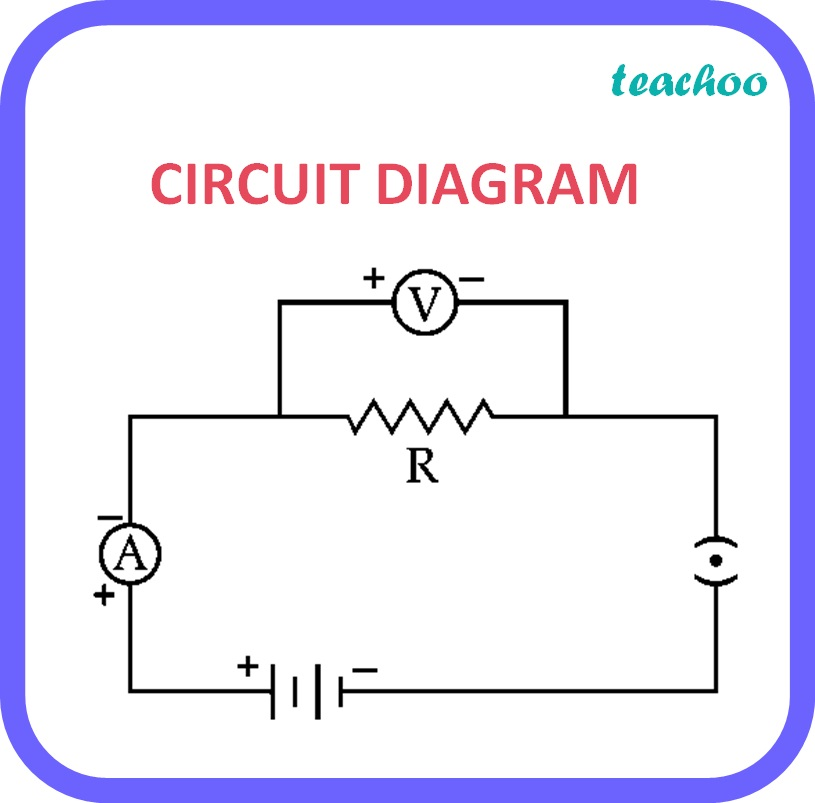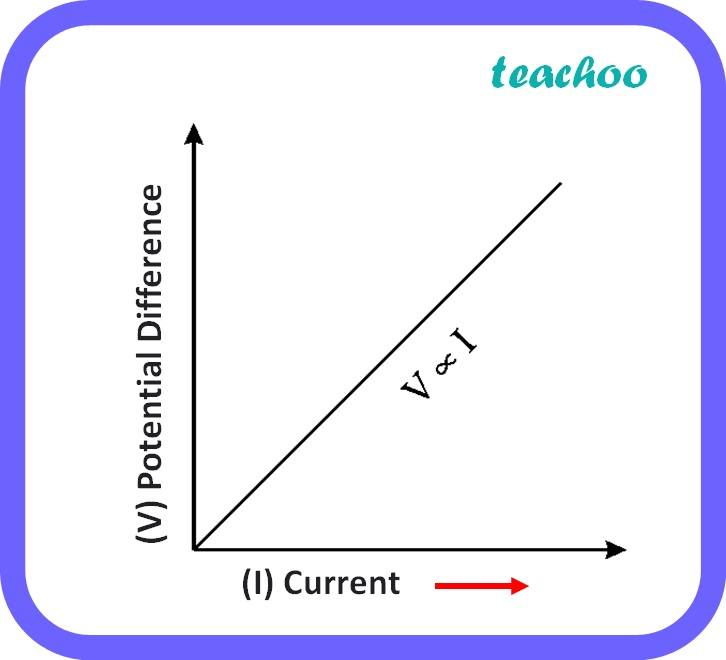Past Year - 5 Mark Questions

Class 10
Chapter 12 Class 10 - Electricity

## (i) Draw a labelled circuit diagram to study a relationship between potential difference (V) across the two ends of a conductor and the current (I) flowing through it. State the formula to show how I in a conductor varies when V across it is increased step wise. Show this relationship also on a schematic graph.

The formula ie., Ohm’s law , states that the current passing through a conductor is directly proportional to the potential difference across its ends, provided the physical conditions like temperature, density etc. remain unchanged.Graph showing relationship between V and I:## (ii) Calculate the resistance of a conductor if the current flowing through it is 0.25

Given,

• I = 0.25 A
• V = 1 V

According to Ohm’s law,

V = IR

Where,

• V is potential difference
• I is electric current
• R is resistance

Therefore, R = V / I

= 1 / 0.25

= 4 ohm

Learn in your speed, with individual attention - Teachoo Maths 1-on-1 Class